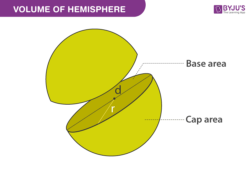# Volume of Hemisphere

In geometry, we have studied different types of three-dimensional shapes. In three-dimensional shapes, the solids have three different measurements or dimensions like length, breadth and height. We know that 3D shapes do not lie on a piece of paper. Usually, most of the three-dimensional objects are obtained from the rotation of the two-dimensional objects.

One of the best examples of the 3D shape is the sphere which is obtained from the rotation of 2D shape called the circle. Our earth is a good example of a sphere which is spherical.

## Hemisphere Definition

A sphere is defined as a set of points in three-dimension, and all the points lying on the surface is equidistant from the centre. When a plane cuts across the sphere at the centre or equal parts, it forms a hemisphere. We can say, a hemisphere is exactly half of a sphere. In general, a sphere makes exactly two hemispheres. One such good example of the hemisphere is our earth. Our earth consists of two hemispheres, namely Southern Hemisphere and the Northern Hemisphere.

## Hemisphere VolumeWe can easily find the volume of the hemisphere since the base of the sphere is circular. The volume of the hemisphere is derived by Archimedes.

The volume of a hemisphere = (2/3)πr3 cubic units.

Where π is a constant whose value is equal to 3.14 approximately.

“r” is the radius of the hemisphere.

## Hemisphere Equation

When the radius “R” is centred at the origin, it is given by

x2 + y2 + z2 = R2

The Cartesian form or equation of a hemisphere with the radius “R” at the point (x0, y0, z0) is written as

(x-x0)2 + (y- y0)2 + (z- z0)2 = R2

Therefore, the spherical coordinates of the hemisphere are given as follows

x = r cos θ sin ∅

y = r sin θ cos ∅

z = r cos ∅

## Solved Problem

A sample problem on hemisphere is given below

### Question:

Find the volume of the hemisphere whose radius is 6 cm.

### Solution:

Given:

The volume of a hemisphere = (2/3)πr3 cubic units.

Substitute the value of r in the formula.

V = (2/3) × 3.14 × 6 × 6 × 6

V = 2× 3.14 × 2 × 6 × 6

V = 452.16

Therefore, the volume of the hemisphere is 452.16 cubic units.

Stay tuned with BYJU’S – The Learning App for many similar topics and also watch videos to learn with ease in an engaging way.

 Related Links Volume Of A Cylinder Volume of A Cone Volume Of Sphere Volume Of A Pyramid

Test your Knowledge on Volume of Hemisphere